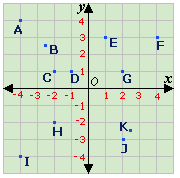## Definition Of Coordinate Plane

Coordinate Plane is a plane formed by the intersection of a horizontal number line with a vertical number line.

The horizontal number line is called the x-axis and the vertical number line is called the y-axis.

The number lines intersect at their zero points. This point of intersection is called the origin and written as (0, 0).

### Solved Example on Coordinate Plane

#### Ques: Name the point having the coordinates (- 2.5, 2.5) on the coordinate plane.A. B
B. E
C. G
D. C